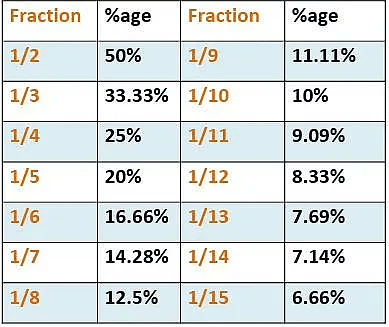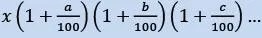# Important Formulas and Tips of Percentage

Percentages

• In mathematics, a percentage is a number or ratio that can be expressed as a fraction of 100. If we have to calculate percent of a number, divide the number by whole and multiply by 100. Hence, the percentage means, a part per hundred. The word percent means per 100. It represented by the symbol “%”.
• Percentages have no dimension. Hence it is called a dimensionless number.
• Percentages can also be represented in decimal or fraction form, such as 0.6%, 0.25%, etc.

Important Formulas

1. Percent implies “for every hundred” – % is read as a percentage and x % is read as x per cent.
2. To calculate:
• p % of y = (p/100) * y = (p * y)/100
• p % of y = y % of p
3. To find what percentage of x is y: y/x * 100
4. To calculate percentage change in value
• Percentage change = {change/(initial value)} x 100
5. Percentage point change = Difference of two percentage figures
6. Increase N by S % = N( 1+ S/100 )
7. Decrease N by S % = N (1 – S/100)
8. If the value of an item goes up/down by x%, the percentage reduction/increment to be now made to bring it back to the original point is 100x/ (100 + x) %.
9. If A is x% more /less than B, then B is 100x/(100 + x) % less/more than A.
10. If the price of an item goes up/down by x %, then the quantity consumed should be reduced by 100x/ (100 + x)% so that the total expenditure remains the same.
11. Successive Percentage Change
• If there are successive percentage increases of a % and b%, the effective percentage increase is: {(a + b + (ab/100)}%

12. Percentage – Ratio Equivalence

Table: Fractions and their percentage equivalents:Percentage Tips

• r% change can be nullified by (100r/100 + r)% change in another direction.
Example: An increase of 25% in prices can be nullified by a reduction of [100 x 25/(100 + 25)] = 20% reduction in consumption.
• If a number ‘x’ is successively changed by a%, b%, c%…
⇒ Final value =• The net change after two successive changes of a% and b% is:Example: What is 27% of 90?
Solution: Our thought process mostly would be 10% of 90 is 9, 5% is 4.5 and 2% is 1.8

hence 27 % of 90 = 18 + 4.5 + 1.8 = 24.3 (5 seconds max).

There would be faster methods but we are not going to any math Olympiad. Practice this method religiously and you will be quick enough for CAT and similar exams.

Example: What is 90% of 27?

Solution: No need to calculate again, we already did it and got the answer as 24.3!

27% of 90 = 27 x 90 / 100 = 90 x 27 / 100 = 90% of 27

That means when we solve one percentage sum we are actually solving two. And this comes very handy if one problem is much easier to solve than the other one.

Example: What would be the final value if 60 is increased by 30%?

Solution: Final value = 60 x (1 + 30/100) = 78

(Else we know 10% of 60 = 6, so 30% increase should add 18 yielding 78 )

Now what would be the final value if 60 is increased by 30% and then by 50%

Final Value = 60 x (1 + 30/100) (1 + 50/100) = 117

Let the successive increase in percentages be a% and b%. In that case, the total increase will be (a + b + ab/100) % (for decrease use a negative sign).

Scroll to Top